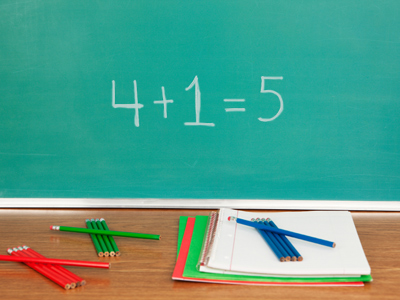To check if 4 + 1 = 5 you could try seeing if 5 - 1 = 4 or 5 - 4 = 1.

# Checking Calculations with Inverse Operations

This Math quiz is called 'Checking Calculations with Inverse Operations' and it has been written by teachers to help you if you are studying the subject at elementary school. Playing educational quizzes is a fun way to learn if you are in the 1st or 2nd grade - aged 6 to 8.

It costs only \$12.50 per month to play this quiz and over 3,500 others that help you with your school work. You can subscribe on the page at Join Us

In order to check a calculation, it is useful to know the inverse (or opposite) operation. For example, the inverse operation of addition is subtraction so to check 5 + 6 = 11, you could try 11 - 6 = 5 or 11 - 5 = 6. Another way of checking an addition calculation would be to add the numbers up in a different order - this of course does not work for subtraction!

Can you check the calculations by adding in a different order or by using the inverse operation?

1.
Which calculation could you use to check that 16 - 9 = 7 is correct?
16 + 9 = 7
9 + 7 = 16
7 - 16 = 9
7 - 9 = 16
If 9 subtracted from 16 leaves 7, then by adding the 9 back again, you should be back to where you started
2.
How do you know that 20 - 10 = 10?
Because I know 10 + 10 = 20
Because it's a subtraction
Using an addition fact can help to solve a subtraction
3.
How would you check 45 - 23 = 22?
By subtracting 22 from 23
By finding the total of all the numbers
Adding the two smaller numbers should give the larger number
4.
How could you check 62 + 38 = 100?
By taking away 100
By finding the difference between 62 and 38
By subtracting 100 from 62
By subtracting 38 from 100
If 62 + 38 = 100 is correct, then 100 - 38 should give an answer of 62
5.
If 29 - 15 = 14, what could you do to check the answer?
Subtract the numbers in a different order
Take out any harder numbers
Put 14 at the beginning of the calculation
You can use addition to check a subtraction
6.
Use number pairs, or bonds, to 20 to find the incorrect calculation:
20 - 13 = 7
20 - 8 = 12
20 - 16 = 14
20 - 2 = 18
16 + 14 gives a total of 30, not 20
7.
What does 'inverse operation' mean?
Doing the calculation upside down
Making a mistake on purpose
The opposite operation
A medical procedure
The inverse of subtraction is addition, the inverse of addition is subtraction
8.
If 3 + 4 + 10 + 3 = 20, what could you do to check the answer?
Take out all the larger numbers
Use multiplication
Add some extra numbers in too
Add the numbers up again in a different order:
10 + 3 + 3 + 4 or 10 + 4 + 3 + 3
Addition can be done in any order
9.
Which calculation could you use to check that 15 + 8 = 23 is correct?
23 + 8 = 15
23 + 15 = 8
15 - 8 = 23
23 - 8 = 15
If a group of 15 and a group of 8 are combined, taking one group away again should leave you with the other
10.
Use number pairs, or bonds, to 20 to find the incorrect calculation:
20 - 10 = 20
20 - 0 = 20
20 - 15 = 5
20 - 19 = 1
20 + 10 would not give a total of 20
Author:  Angela Smith